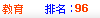Sclub交友聊天~加入聊天室當版主# [函数] 一个关于导数的问题

 f(x)是一个定义在R上的连续函数并且可导 集合A由f(x)上任意不同两点连线的斜率组成 集合B由f(x)上的点的切线的斜率组成 试问f(x)需要什么条件可以使A=B -------------------------------------------- 注意：分别有两个例子 1:f(x)=x^3+x A≠B 2:f(x)=x^2 A=B --------------------------

 导函数连续且无界
 如果要满足A=B，那么必为其中之一： (1). 若f ''(x)存在，则f ''(x)恒大于0，或f ''(x)恒小于0； (2). 若存在t∈(a,b), s∈(b,c),且f ''(t)恒大于0，f ''(s)恒小于0，或f ''(t)恒小于0，f ''(s)恒大于0，那么x=b叫做f(x)的拐点，若存在拐点x=b, 则必存在c≠b，满足f '(c)=f '(b)，且x=c不是拐点. 例如: f(x)=x^3+x,  存在拐点x=0, f '(0)=1, 但不存在c≠0，使得f '(c)=1.  则A≠B. f(x)=x^2, 不存在拐点，f ''(x)=2,恒大于0. 则A=B.
 拐点是f '(x)的极值点~